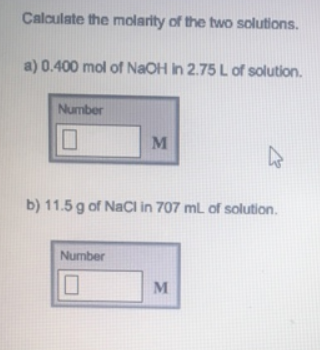Chemistry Molarity Solution: Calculate the molarity of the two solutions.a. 0.4...

# Solution: Calculate the molarity of the two solutions.a. 0.400 mol of NaOH in 2.75 L of solution.b. 11.5 g of NaCl in 707 mL of solution.

Problem

Calculate the molarity of the two solutions.

a. 0.400 mol of NaOH in 2.75 L of solution.

b. 11.5 g of NaCl in 707 mL of solution.View Complete Written Solution